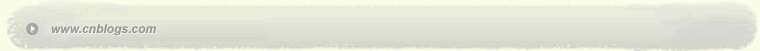Impossible is nothing 爱过知情重醉过知酒浓 　　花开花谢终是空 　　缘份不停留像春风来又走 　　女人如花花似梦

 < 2023年3月 >
2627281234
567891011
12131415161718
19202122232425
2627282930311
2345678

• 随笔 - 8
• 文章 - 91
• 评论 - 16
• 引用 - 0

# 相册

•

### 评论排行榜

1 下面程序有什么错误：
class base{
private: int i;
public: base(int x){i=x;}
};
class derived: public base{
private: int i;
public: derived(int x, int y) {i=x;}
void printTotal() {int total = i+base::i;}
};

2. Assume you have a class like

class erp
{
HR* m_hr;
FI* m_fi;
public:
erp()
{
m_hr = new HR();
m_fi = new FI();
}
~erp()
{
}
};

if "new FI()" failed in the constructor, how can you detect this problem and
release the properly allocated member pointer m_hr?

3. Check the class and variable definition below:

#include <iostream>
#include <complex>
using namespace std;
class Base
{
public:
Base() { cout<<"Base-ctor"<<endl; }
~Base() { cout<<"Base-dtor"<<endl; }
virtual void f(int) { cout<<"Base::f(int)"<<endl; }
virtual void f(double) {cout<<"Base::f(double)"<<endl; }
virtual void g(int i = 10) {cout<<"Base::g()"<<i<<endl; }
};

class Derived: public Base
{
public:
Derived() { cout<<"Derived-ctor"<<endl; }
~Derived() { cout<<"Derived-dtor"<<endl; }
void f(complex<double>) { cout<<"Derived::f(complex)"<<endl; }
virtual void g(int i = 20) {cout<<"Derived::g()"<<i<<endl; }
};

Base b;
Derived d;

Base* pb = new Derived;
Select the correct one from the four choices:
Cout<<sizeof(Base)<<endl;
A. 4 B.32 C.20 D.Platform-dependent
Cout<<sizeof(Base)<<endl;
A. 4 B.8 C.36 D.Platform-dependent
pb->f(1.0);
A.Derived::f(complex) B.Base::f(double)
pb->g();
A.Base::g() 10 B.Base::g() 20
C.Derived::g() 10 D.Derived::g() 20

4.Implement the simplest singleton pattern(initialize if necessary).

5.Name three sort algorithms you are familiar with. Write out the correct
order by the average time complexity.

6.Write code to sort a duplex direction linklist. The node T has overridden
the comparision operators.

7.Below is usual way we find one element in an array:

const int *find1(const int* array, int n, int x)
{
const int* p = array;
for(int i = 0; i < n; i++)
{
if(*p == x)
{
return p;
}
++p;
}
return 0;
}

In this case we have to bear the knowledge of value type "int", the size of
array, even the existence of an array. Would you re-write it using template
to eliminate all these dependencies?
posted on 2006-04-12 22:35 笑笑生 阅读(1322) 评论(2)  编辑 收藏 引用 所属分类: STL/BOOST• # re: sap的几道笔试题目(转)  任我行 Posted @ 2006-04-13 08:47
1.
public: derived(int x, int y) {i=x;}
//derived(int x, int y):base(y){i=x;}
void printTotal() {int total = i+#base::i#;}
2.
try{
m_fi = new FI();
}
delete m_hr;
}

3.前两题题目重复吧。
Cout<<sizeof(Base)<<endl; //32
A. 4 B.32 C.20 D.Platform-dependent
Cout<<sizeof(Base)<<endl;
A. 4 B.8 C.36 D.Platform-dependent
3.3
pb->f(1.0); //Base::f(double)
pb->g();//Derived::g()20  回复  更多评论

• # re: sap的几道笔试题目(转)  tuesday Posted @ 2006-04-13 19:39
第二题可以这样做
template<class T>
class PWrap
{
T* ptr;
PWrap() { ptr = new T;}
~PWrap() {delete ptr;}
};

class erp
{
PWrap<HR> m_hr;
PWrap<FI> m_fi;
};  回复  更多评论Copyright © 笑笑生 Powered by: 博客园 模板提供：沪江博客